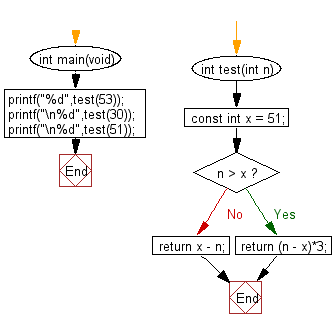﻿ C exercises: Get the absolute difference between n and 51 - w3resource# C Exercises: Get the absolute difference between n and 51

## C-programming basic algorithm: Exercise-2 with Solution

Write a C program to get the absolute difference between n and 51. If n is greater than 51 return triple the absolute difference.

C Code:

``````#include <stdio.h>
int main(void){
printf("%d",test(53));
printf("\n%d",test(30));
printf("\n%d",test(51));
}

int test(int n)
{
const int x = 51;

if (n > x)
{
return (n - x)*3;
}
return x - n;
}
``````

Sample Output:

```6
21
0
```

Pictorial Presentation:Flowchart: